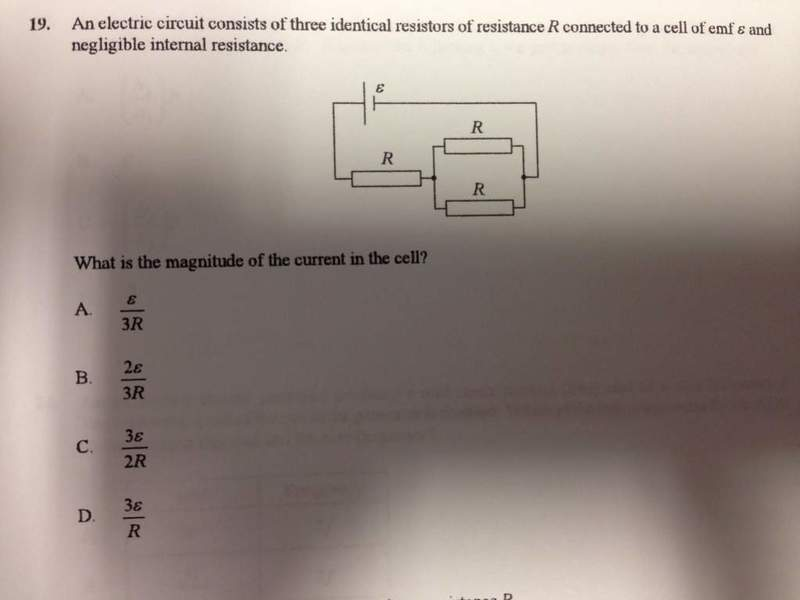# Current in the cellTrying to work out how to do this. I know the answer is B but don't know how.

Internal resistance is negligible so I assume I just use I =V/R. I've tried using sample values for R eg 5 and V =10V giving I as 1.3 but am no closer to 2/3. What am I missing?

nasu
Gold Member
Can you show how did you do it with sample values? How do you calculate the current?

If R=5ohms

1/5+1/5=0.4

1/0.4 = 2.5 ohms in parallel

2.5 + 5 = 7.5 ohms total

If v is 10v

I = v/R = 10/7.5 = 1.333333

nasu
Gold Member
And this is compatible with answer b, isn't it?
Try to calculate 2e/3R. How much is it?

You have the right idea. Now just do the same thing with symbols rather than numbers.
What is the equivalent resistance, in terms of R?

Got it! Many thanks